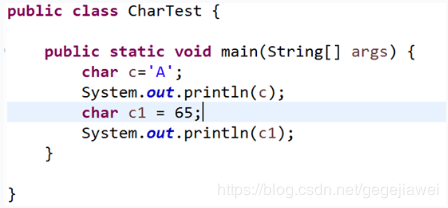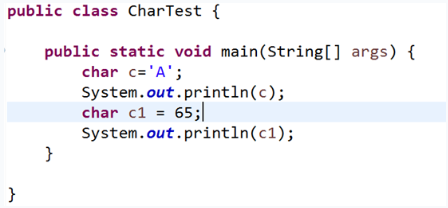• 1、下列属于合法 Java 标识符是？（多选）ABF A. $value B. Void C. class D. 1abc E. my value F.void_class 2、下列哪一个选项不是Java关键字？C A. class B. package C. Void D. static 3、下列可以表示数值... 选择题 1、下列属于合法的 Java 标识符是？（多选）ABF A.$value
B. Void
C. class
D. 1abc
E. my value
F.void_class
2、下列哪一个选项不是Java的关键字？C
A. class
B. package
C. Void
D. static
3、下列可以表示数值型数据的数据类型是？（多选）ABD
A. byte
B. float
C. boolean
D. long
4、关于数据类型的说法错误的是？B
A. 数据类型分为基本数据类型和引用数据类型
B. 数组属于基本数据类型
C. 类属于引用数据类型
D. int和double属于基本数据类型
5、下列关于赋值运算符的说法正确的是？(多选) AC
A. 使用“=”给变量进行赋值
B. 使用“==”给变量进行赋值
C. "="叫作赋值运算符，将运算符右边的值赋给左边的变量
D. "="叫作赋值运算符，将运算符左边的值赋给右边的变量
6、以下对double类型字面值的表示错误的是？D
A. 1.23
B. 1.23d
C. 1.23D
D.1.23f
7、以下语句哪个是错误的？D
A. double d=12.3d;
B. double d=12.3D;
C. float f=1.23f;
D.float f=1.23;
8、下面代码运行后的结果为? DA. A
B. 65
C. A 65
D.A A
9、以下字符型字面值中，不合法的是? C
A. ‘@’
B. ‘&’
C. “M”
D.‘课’
A. “”
B. ‘ab’
C. ‘a’
D."\u0067"
11、以下哪两条语句是正确的？（多选）CD
A. char ch=65539;
B. int n=5.6f;
C. double d=1.2f;
D. double d=1.23;float f=(float)d；
**
编程题
**
1、分别定义float、double、int类型的数据，并打印输出。
public class Test1 {
public static void main(String[] args) {
// 定义一个float类型的变量f1，值为98.4
float f1 = 98.4F;
//定义一个float类型的变量f2，将f1的值赋值给f2
float f2 = f1;
//定义一个整型变量n，值为55
int n = 55;
//定义一个double类型的变量d1，值为555.3
double d1 = 555.3;
//将n的值赋值给d1
d1 = n;
//打印输出
System.out.println(f1);//98.4
System.out.println(f2);//98.4
System.out.println(n);//55
System.out.println(d1);//55.0
}
}

2、定义字符型变量，赋值后输出。
public class Test2 {
public static void main(String[] args) {
// 定义字符变量c，并赋值为'S'
char c = 'S';
//输出c的值
System.out.println(c);//S
//定义字符变量c1，并赋值为78
char c1 = 78;
System.out.println(c1);//N
}
}

3、定义布尔类型和字符串类型的变量，为它们赋值，并打印输出。
public class Test3 {
public static void main(String[] args) {
// 定义一个布尔类型的变量flag，赋值为true，并输出
boolean flag = true;
System.out.println(flag);//true
//定义一个字符串类型的变量str，值为"hello world"，并输出
String str = "hello world";
System.out.println(str);//hello world
//定义一个字符串类型的变量str1，值为空串
String str1 = "";
//将str的值赋值给str1，并输出
str1 = str;
System.out.println(str1);//hello world
}
}

4、定义各种数据类型的变量，为它们赋值，并打印输出。
public class Test4 {
public static void main(String[] args) {
// 定义一个整型变量n，值为98
int n = 98;
//定义一个char类型的变量ch，将变量n赋值给ch，然后输出ch的值
char ch = (char) n;
System.out.println(ch);//b
//定义一个长整型变量var，值为190000000，并输出
long var = 190000000;
System.out.println(var);//190000000
//定义一个double类型的变量d，将变量var的值赋值给d
double d = var;
System.out.println(d);//1.9E8
}
}

5、定义各种数据类型的变量，按要求为它们赋值，并打印输出。
public class Test5 {
public static void main(String[] args) {
//将一个整型字面值67832赋值给char类型变量c
//并将c的值输出
char c = (char) 67832;
System.out.println(c);
//定义一个整型变量n，值为65
int n = 65;
//定义一个字符型变量c1，赋值为n，并输出c1的值
char c1 = (char) n;
System.out.println(c1);//A
//定义一个长整型变量l，值为987654321
long l = 987654321L;
//定义一个整型变量i，赋值为l，并输出i的值
int i = (int) l;
System.out.println(i);//987654321
//定义一个float类型变量f，将变量l的值赋值给f，并输出f的值
float f = l;
System.out.println(f);//9.8765434E8
//将float的值f，重新赋值给变量l，并输出l的值
l = (long) f;
System.out.println(l);//987654336
}
}



展开全文• 下列属于合法 Java 标识符是？（多选）ABF A. $value B. Void C. class D. 1abc E. my value F.void_class 下列哪一个选项不是Java关键字？C A. class B. package C. Void D. static 下列可以表示... 一、选择 下列属于合法的 Java 标识符是？（多选）ABF A.$value  B. Void  C. class  D. 1abc  E. my value  F.void_class


下列哪一个选项不是Java的关键字？C
A. class  B. package  C. Void  D. static


下列可以表示数值型数据的数据类型是？（多选）ABD
A. byte  B. float  C. boolean D. long


关于数据类型的说法错误的是？B
A. 数据类型分为基本数据类型和引用数据类型
B. 数组属于基本数据类型
C. 类属于引用数据类型
D. int和double属于基本数据类型


下列关于赋值运算符的说法正确的是？(多选)AC
A. 使用“=”给变量进行赋值
B. 使用“==”给变量进行赋值
C. "="叫作赋值运算符，将运算符右边的值赋给左边的变量
D. "="叫作赋值运算符，将运算符左边的值赋给右边的变量


以下对double类型字面值的表示错误的是？D
A. 1.23  B. 1.23d  C. 1.23D D.1.23f


以下语句哪个是错误的？D
A. double d=12.3d;  B. double d=12.3D;  C. float f=1.23f; D.float f=1.23;


下面代码运行后的结果为?D
A. A   B. 65  C. A 65  D.A A


以下字符型字面值中，不合法的是?C
A. '@'   B. '&'  C. "M"  D.'课'


A. ""  B. 'ab' C.   'a'  D."\u0067"


以下哪两条语句是正确的？（多选）CD
A. char ch=65539;  B. int n=5.6f; C. double d=1.2f;  D. double d=1.23;float f=(float)d；


二、编程

分别定义float、double、int类型的数据，并打印输出。
public class FloatDemo {
public static void main(String[] args) {
// 定义一个float类型的变量f1，值为98.4
float f1 = 98.4f;
System.out.println(f1);
//定义一个float类型的变量f2，将f1的值赋值给f2
float f2 = f1;
System.out.println(f2);
//定义一个整型变量n，值为55
int n = 55;
System.out.println(n);
//定义一个double类型的变量d1，值为555.3
double d1 = 555.3;
System.out.println(d1);
//将n的值赋值给d1
d1 = n;
System.out.println(d1);
}
}


定义字符型变量，赋值后输出。
public class CharTest {

public static void main(String[] args) {
// 定义字符变量c，并赋值为'S'
char c = 'S';
//输出c的值
System.out.println(c);
//定义字符变量c1，并赋值为78
char c1 = 78;
//输出c1的值
System.out.println(c1);
}
}


定义布尔类型和字符串类型的变量，为它们赋值，并打印输出。
public class StringDemo {
public static void main(String[] args) {
// 定义一个布尔类型的变量flag，赋值为true，并输出
boolean flag = true;System.out.println(flag);
//定义一个字符串类型的变量str，值为"hello world"，并输出
String str = "hello world";System.out.println(str);
//定义一个字符串类型的变量str1，值为空串
String str1 = "";
//将str的值赋值给str1，并输出
str1 = str;
System.out.println(str1);
}
}


定义各种数据类型的变量，为它们赋值，并打印输出。
public class TypeExchange {
public static void main(String[] args) {
// 定义一个整型变量n，值为98
byte n = 98;
//定义一个char类型的变量ch，将变量n赋值给ch，然后输出ch的值
char ch = (char)n;
System.out.println(ch);
//定义一个长整型变量var，值为190000000，并输出
long var = 190000000L;
System.out.println(var);
//定义一个double类型的变量d，将变量var的值赋值给d
double d = var;
System.out.println(d);
}
}


定义各种数据类型的变量，按要求为它们赋值，并打印输出。
public class TypeExchange {
public static void main(String[] args) {
// 将一个整型字面值67832赋值给char类型变量c
//并将c的值输出
char c = (char)67832;
System.out.println(c);
//定义一个整型变量n，值为65
byte n = 65;
//定义一个字符型变量c1，赋值为n，并输出c1的值
char c1 = (char)n;
System.out.println(c1);
//定义一个长整型变量l，值为987654321
long l = 987654321L;
//定义一个整型变量i，赋值为l，并输出i的值
int i = (int)l;
System.out.println(i);
//定义一个float类型变量f，将变量l的值赋值给f，并输出f的值
float f = l;
System.out.println(f);
//将float的值f，重新赋值给变量l，并输出l的值
l = (long)f;
System.out.println(l);
}
}

展开全文• 1.下列属于合法Java标识符是?(多选) A.$valueB.VoldC.classD.1abcE.myvalueF.void_class ABF 2.下列哪一一个选项不是Java关键字? A.classB.packageC.VoidD.static C 3.下列可以表示数值型数据数据类型是?(多选... 1.下列属于合法的Java标识符是?(多选) A.$valueB.VoldC.classD.1abcE.myvalueF.void_class
ABF
2.下列哪一一个选项不是Java的关键字?
A.classB.packageC.VoidD.static
C
3.下列可以表示数值型数据的数据类型是?(多选)
A.byteB.floatC.booleanD.long
4.关于数据类型的说法错误的是?
A.数据类型分为基本数据类型和引用数据类型
B.数组属于基本数据类型
C.类属于引用数据类型
D.int和double属于基本数据类型
B
5.下列关于赋值运算符的说法正确的是?(多选)
A.使用“=”给变量进行赋值
B.使用“==”给变量进行赋值
C.“=”叫作赋值运算符，将运算符右边的值赋给左边的变量
D.“=”叫作赋值运算符，将运算符左边的值赋给右边的变量
AC
6.以下对double类型字面值的表示错误的是?
A.1.23B.1.23dC.1.23DD.1.23f
D
7.以下语句哪个是错误的?
A.doubled=12.3d;B.doubled=12.3D;C.floatf=1.23f;D.floatf=1.23;
D
8.下面代码运行后的结果为?
publicclassCharTest{
publicstaticvoidmain(String[]args){
charc=‘A’;
charc1=65;|
System.out.println(c1);
}
}
A.AB.65C.A65D.AA
D
9.以下字符型字面值中，不合法的是?
A.’@‘B.’&’C.“M”D.‘课’
A
10.以下哪两个是合法的字符串字面值?(多选)
A.“”B.'ab’C.'a’D."\u0067"
11.以下两条语句是正确的?(多选)
A.charch=65539;B.intn=5.6f;C.doubled=1.2f;D.doubled=1.23;float
CD
二、编程

分别定义float、double、int类型的数据，并打印输出。
public class FloatDemo {     public static void main(String[] args) {
// 定义一个float类型的变量f1，值为98.4
//定义一个float类型的变量f2，将f1的值赋值给f2
//定义一个整型变量n，值为55
//定义一个double类型的变量d1，值为555.3
//将n的值赋值给d1     } }2. 定义字符型变量，赋值后输出。
public class CharTest {
public static void main(String[] args) {   // 定义字符变量c，并赋值为’S’

//输出c的值
//定义字符变量c1，并赋值为78
//输出c1的值
}
}3. 定义布尔类型和字符串类型的变量，为它们赋值，并打印输出。
public class StringDemo {     public static void main(String[] args) {
// 定义一个布尔类型的变量flag，赋值为true，并输出
//定义一个字符串类型的变量str，值为"hello world"，并输出
//定义一个字符串类型的变量str1，值为空串
//将str的值赋值给str1，并输出
} }4. 定义各种数据类型的变量，为它们赋值，并打印输出。
public class TypeExchange {     public static void main(String[] args) {
// 定义一个整型变量n，值为98
//定义一个char类型的变量ch，将变量n赋值给ch，然后输出ch的值
//定义一个长整型变量var，值为190000000，并输出
//定义一个double类型的变量d，将变量var的值赋值给d     } }5. 定义各种数据类型的变量，按要求为它们赋值，并打印输出。
public class TypeExchange {     public static void main(String[] args) {
// 将一个整型字面值67832赋值给char类型变量c
//并将c的值输出      //定义一个整型变量n，值为65
//定义一个字符型变量c1，赋值为n，并输出c1的值
//定义一个长整型变量l，值为987654321
//定义一个整型变量i，赋值为l，并输出i的值
//定义一个float类型变量f，将变量l的值赋值给f，并输出f的值
//将float的值f，重新赋值给变量l，并输出l的值    } }展开全文• 1.下列属于合法 Java 标识符是？（多选） ABF A. $value B. Void C. class D. 1abc E. my value F.void_class 2.下列哪一个选项不是Java关键字？C A. class B. package C. Void D. static 3.下列可以表示数值型... 一、选择 1.下列属于合法的 Java 标识符是？（多选） ABF A.$value B. Void C. class D. 1abc E. my value F.void_class
2.下列哪一个选项不是Java的关键字？C
A. class B. package C. Void D. static
3.下列可以表示数值型数据的数据类型是？（多选）ABD
A. byte B. float C. boolean D. long
4.关于数据类型的说法错误的是？B
A. 数据类型分为基本数据类型和引用数据类型
B. 数组属于基本数据类型
C. 类属于引用数据类型
D. int和double属于基本数据类型
5.下列关于赋值运算符的说法正确的是？(多选)AC
A. 使用“=”给变量进行赋值
B. 使用“==”给变量进行赋值
C. "="叫作赋值运算符，将运算符右边的值赋给左边的变量
D. "="叫作赋值运算符，将运算符左边的值赋给右边的变量
6.以下对double类型字面值的表示错误的是？D
A. 1.23 B. 1.23d C. 1.23D D.1.23f
7.以下语句哪个是错误的？D
A. double d=12.3d; B. double d=12.3D; C. float f=1.23f; D.float f=1.23;
8.下面代码运行后的结果为?D
A. A B. 65 C. A 65 D.A A9.以下字符型字面值中，不合法的是?C
A. ‘@’ B. ‘&’ C. “M” D.‘课’
A. “” B. ‘ab’ C. ‘a’ D."\u0067"
11.以下哪两条语句是正确的？（多选）CD
A. char ch=65539; B. int n=5.6f; C. double d=1.2f; D. double d=1.23;float f=(float)d；
二、编程
1、分别定义float、double、int类型的数据，并打印输出。
package my_first_project;

public class FloatDemo {
public static void main(String[] args){
// 定义一个float类型的变量f1，值为98.4
float f1 = 98.4f;
//定义一个float类型的变量f2，将f1的值赋值给f2
float f2 = f1;
f2 = f1;
//定义一个整型变量n，值为55
int n = 55;
//定义一个double类型的变量d1，值为555.3
double d1 = 555.3;
//将n的值赋值给d1
d1 = n;
System.out.println(f1);
System.out.println(f2);
System.out.println(n);
System.out.println(d1);
}
}

2、定义字符型变量，赋值后输出。
package my_first_project;

public class CharTest {
public static void main(String[] args) {
// 定义字符变量c，并赋值为'S'
char c = 's';

//输出c的值
System.out.println(c);

//定义字符变量c1，并赋值为78
char c1 = 78;

//输出c1的值
System.out.println(c1);
}
}

3、定义布尔类型和字符串类型的变量，为它们赋值，并打印输出。
package my_first_project;

public class StringDemo {
public static void main(String[] args) {
// 定义一个布尔类型的变量flag，赋值为true，并输出
boolean flag = true;
//定义一个字符串类型的变量str，值为"hello world"，并输出
String str = "hello world";
System.out.println(str);
//定义一个字符串类型的变量str1，值为空串
String str1 = "";
//将str的值赋值给str1，并输出
str1 = str;
System.out.println(str1);
}
}

4、定义各种数据类型的变量，为它们赋值，并打印输出。
package my_first_project;

public class TypeExchange {
public static void main(String[] args) {
// 定义一个整型变量n，值为98
int n = 98;
//定义一个char类型的变量ch，将变量n赋值给ch，然后输出ch的值
char ch = (char)n;
System.out.println(ch);
//定义一个长整型变量var，值为190000000，并输出
long var = 190000000L;
System.out.println(var);
//定义一个double类型的变量d，将变量var的值赋值给d
double d = var;
System.out.println(d);
}
}

5、定义各种数据类型的变量，按要求为它们赋值，并打印输出。
package my_first_project;

public class TypeExchang {
public static void main(String[] args) {
// 将一个整型字面值67832赋值给char类型变量c
//并将c的值输出
char c = (char)67832;
System.out.println(c);
//定义一个整型变量n，值为65
int n = 65;
//定义一个字符型变量c1，赋值为n，并输出c1的值
char c1 = (char)n;
System.out.println(c1);
//定义一个长整型变量l，值为987654321
long l = 987654321L;
//定义一个整型变量i，赋值为l，并输出i的值
int i = (int)l;
System.out.println(i);
//定义一个float类型变量f，将变量l的值赋值给f，并输出f的值
float f = l;
System.out.println(f);
//将float的值f，重新赋值给变量l，并输出l的值
l = (long)f;
System.out.println(l);
}
}



展开全文• 下列属于合法 Java 标识符是？（多选）ABF A. $value B. Void C. class D. 1abc E. my value F.void_class 下列哪一个选项不是Java关键字？ C A. class B. package C. Void D. static 下列可以表示数值型数据...java • 1.下列属于合法 Java 标识符是？（多选）ABF A.$value B. Void C. class D. 1abc E. my value F.void_class 2.下列哪一个选项不是Java关键字？B A. class B. package C. Void D. static 3.下列可以表示数值型...java
• 1、选择下列属于合法 Java 标识符是？（ABF） A. $value B. Void C. class D. 1abc E. my value F.void_class 2、下列哪一个选项不是Java关键字？（C） A. class B. package C. Void D. static 3、下列可以表示...java • 下列属于合法 Java 标识符是？（bc） A.$value B. Void C. class D. 1abc E. my value F.void_class 下列哪一个选项不是Java关键字？（b） A. class B. package C. Void D. static 下列可以表示数值型数据...
• 下列属于合法 Java 标识符是？（多选）ABF A. $value B. Void C. class D. 1abc E. my value F.void_class 下列哪一个选项不是Java关键字？C A. class B. package C. Void D. static 下列可以表示数值型数据... • 1、下列属于合法 Java 标识符是？（多选） A.$value B. Void C. class D. 1abc E. my value F.void_class 2、下列哪一个选项不是Java关键字？ A. class B. package C. Void D. static 3、下列可以表示数值型...java
• 1.下列属于合法 Java 标识符是？（多选）AF A. $value B. Void C. class D. 1abc E. my value F.void_class 2.下列哪一个选项不是Java关键字？B A. class B. package C. Void D. static 3.下列可以表示数值型...java • 下列属于合法 Java 标识符是？（多选） ABF A.$value B. Void C. class D. 1abc E. my value F.void_class 下列哪一个选项不是Java关键字？C A. class B. package C. Void D. static 下列可以表示数值型数据...java
• 下列属于合法 Java 标识符是？（多选）ABF A. $value B. Void C. class D. 1abc E. my value F.void_class 下列哪一个选项不是Java关键字？C A. class B. package C. Void D. static 下列可以表示数值型数据... • 1.下列属于合法 Java 标识符是？（多选） ABF A.$value B. Void C. class D. 1abc E. my value F.void_class 2.下列哪一个选项不是Java关键字？C A. class B. package C. Void D. static 3.下列可以表示数值型...
• 下列属于合法 Java 标识符是？（多选）A,B,F A. $value B. Void C. class D. 1abc E. my value F.void_class 下列哪一个选项不是Java关键字？C A. class B. package C. Void D. static 下列可以表示数值型数据...java javase • 下列属于合法 Java 标识符是？（多选）ABF A.$value B. Void C. class D. 1abc E. my value F.void_class 下列哪一个选项不是Java关键字？C A. class B. package C. Void D. static 下列可以表示数值型数据...java
• 1.下列属于合法 Java 标识符是？（多选）ABF A. $value B. Void C. class D. 1abc E. my value F.void_class 2.下列哪一个选项不是Java关键字？C A. class B. package C. Void D. static 3.下列可以表示数值型... • 1. 下列属于合法 Java 标识符是？ABF A.$value B. Void C. class D. 1abc E. my value F.void_class 2. 下列哪一个选项不是Java关键字？C A. class B. package C. Void D. static 3. 下列可以表示数值型数据...java
• 下列属于合法 Java 标识符是？（多选） A. \$value B. Void C. class D. 1abc E. my value F.void_class 选择：ABF 下列哪一个选项不是Java关键字？ A. class B. package C. Void D. static 选择：C 下列可以...java
• 迄今为止计算机程序设计语言发展经历了机器语言、汇编语言、高级语言等阶段，C++语言是一种面向对象编程语言，也属于高级语言。 1-2 面向对象编程语言有哪些特点？ 解： 面向对象编程语言与以往各种编程...课后习题答案
• 20.设 i,j 为类 x 中定义的 double 变量名，下列 x 类的构造函数中不正确的是( )。 A) double x(double k ){ i=k; return i; } B) x(double m, double n ){ i=m; j=n; } C) x( ){i=0;j=0 } D) x(double k ){ i=k;...Java
• 下面不属于基本类型的是：c (选择1项) A) boolean B) long C) String D) byte 题目2：d 如下程序中： （1）public class CharToInt （2）{ （3） public static void main(String[] args) （4） { （5） int a,b=...
• 4.2.6　常量指针和指向常量的指针 164 4.2.7　指针和数组 165 4.3　动态内存分配 172 4.3.1　堆的别名——自由存储器 172 4.3.2　new和delete运算符 172 4.3.3　为数组动态分配内存 173 4.3.4　多维数组的...